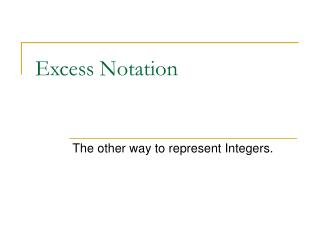# Excess Notation - PowerPoint PPT PresentationDownload PresentationExcess Notation

Excess Notation
Download Presentation## Excess Notation

- - - - - - - - - - - - - - - - - - - - - - - - - - - E N D - - - - - - - - - - - - - - - - - - - - - - - - - - -
##### Presentation Transcript

1. Excess Notation The other way to represent Integers.

2. Excess Notation(examples are in 8 bits to save space) • Fixed length notation system. • Uses 0 to represent negative values. The largest non-negative value: 11111111 The smallest non-negative value: 10000000 The largest negative value is: 01111111 The smallest negative value is: 00000000

3. Excess Notation Consider the 8 patterns in 3 bits:

4. Excess Notation Interpreted as Natural Numbers:

5. Excess Notation Interpreted as Integers in 2’s Complement:

6. Excess Notation Interpreted as Integers in Excess Notation:

7. Excess Notation Three different Interpretations:

8. Excess Notation(examples are in 8 bits to save space) To better understand how binary patterns unpack under the 3 notations, let’s look at an example. Consider the pattern 10111001 Show the value represented if the pattern is: • an unsigned integer • an integer, in 2’s Complement Notation • an integer, in Excess Notation

9. Excess Notation(examples are in 8 bits to save space) The pattern (10111001) has 2 parts:

10. Excess Notation(examples are in 8 bits to save space) The pattern (10111001) has 2 parts: • the MSB

11. Excess Notation(examples are in 8 bits to save space) The pattern (10111001) has 2 parts: • the MSB • the rest

12. Excess Notation(examples are in 8 bits to save space) The pattern (10111001) has 2 parts: • the MSB • the rest Let’s look at the “rest”: 0111001

13. Excess Notation(examples are in 8 bits to save space) The pattern (10111001) has 2 parts: • the MSB • the rest 0111001 represents the Natural number32 + 16 + 8 + 1= 57

14. Excess Notation(examples are in 8 bits to save space) The pattern (10111001) is, therefore, 57 greater than10000000– regardless of the meaning of the MSB.

15. Excess Notation(examples are in 8 bits to save space) As a Natural number,10000000 is 128

16. Excess Notation(examples are in 8 bits to save space) As a Natural number,10000000 is 128 In 2’s Complement,10000000 isthe smallest, negative value… -128

17. Excess Notation(examples are in 8 bits to save space) As a Natural number,10000000 is 128 In 2’s Complement,10000000 isthe smallest, negative value… -128 In Excess Notation,10000000 isthe smallest, non-negative value… 0

18. Excess Notation(examples are in 8 bits to save space) So the pattern 10111001 is 57 greater than: 128 if it’s natural (57+128=185) -128 if it’s 2’s Complement (57-128=-71) 0 if it’s Excess (57+ 0= 57)